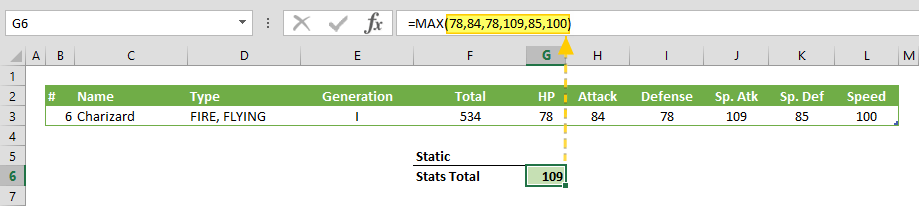The Excel MAX function returns the maximum value in an array or range of cells. In this guide, we’re going to show you how to use the MAX function and also throw in some tips and error handling methods.

# Supported versions

• All Excel versions

# Excel MAX Function Syntax

MAX(number1,[number2],...)

# Arguments

 number1 The first number or numbers where you want to find the maximum. You can enter a number like 4, a cell reference like B6, or a cell range like B2:B8. [number2] Optional. The second number or numbers up to 255 numbers.

Examples

## Static values

=MAX(78,84,78,109,85,100)
formula returns the maximum number in the arguments.## Individual cells

=MAX(G3,H3,I3,J3,K3,L3)
formula evaluates all values in the cells.## Range

=MAX(G3:L3)
formula looks up the values of cells in the range reference. Any valid range can be used with the MAX function.# Tips

• The Excel MAX function ignores string values. However, keep in mind that date or time values are actually processed as numbers in Excel, not strings.
• Use the MAXIFS function to find the maximum number that meets specified conditions.
• The opposite of this function is the MIN function that returns the minimum number in an array.

# Issues

## Error

If there is an error in referred cells or ranges, the MAXfunction returns the same error.